16.2 Boosting 和正则化路径¶

带惩罚的回归¶

Recall

$J(\alpha)$ 是关于系数的函数，并且一般惩罚较大的值．比如

weiya 注：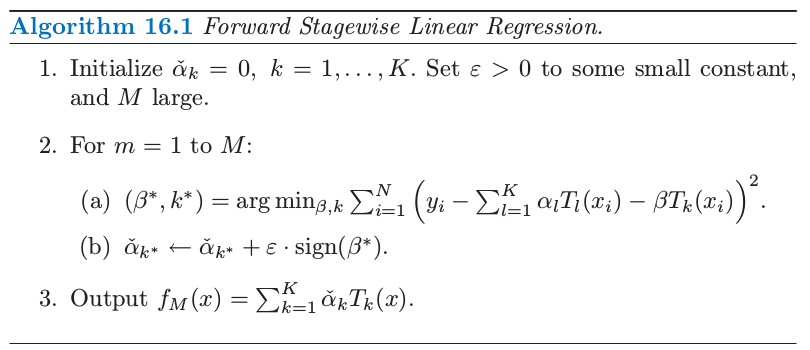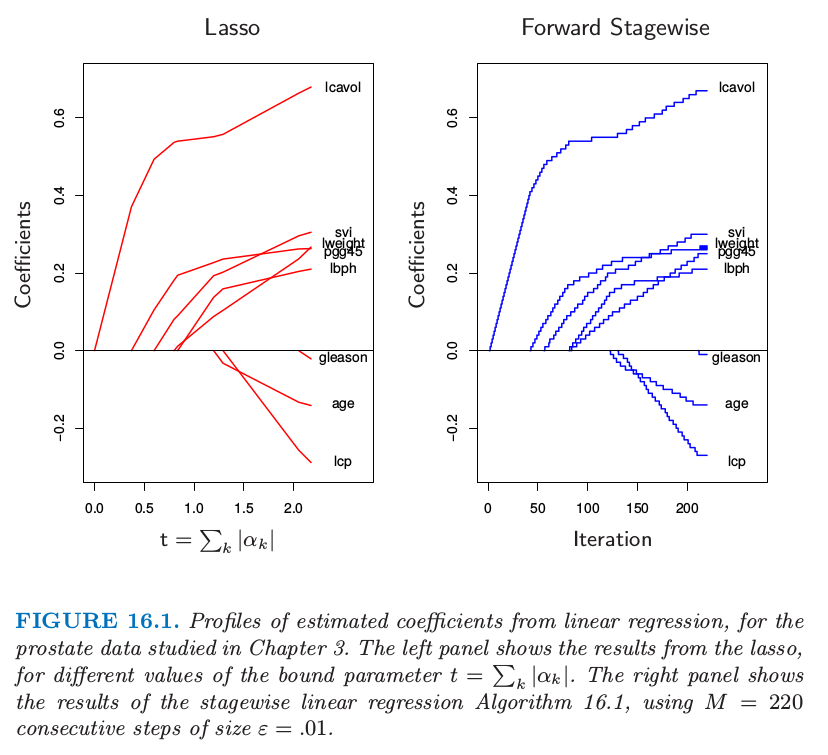Efron et al. (2004)1通过在 $\varepsilon$ 极限的情形下特征化解的路径，精确地描述了这种联系．他们证明对于 lasso 和向前逐步法，系数的路径都是分段线性函数．这能够帮助提出高效的算法，使得整个路径计算时仅用到单个最小二乘拟合相同的花费．这个 最小角回归 (least angle regression) 算法在 3.8.1 节有更多的细节描述．

Hastie et al. (2007)2证明了这个无穷小量向前逐步算法（$FS_0$）拟合了 lasso 的单调版本，在给定系数路径的 角长度 (arc length) 的增长下，最优化了每一步的损失函数（参见 16.2.3 节3.8.1 节）．对于 $\epsilon > 0$ 的情形角长度为 $M\epsilon$，也因此与步数成比例．

weiya 注：Recall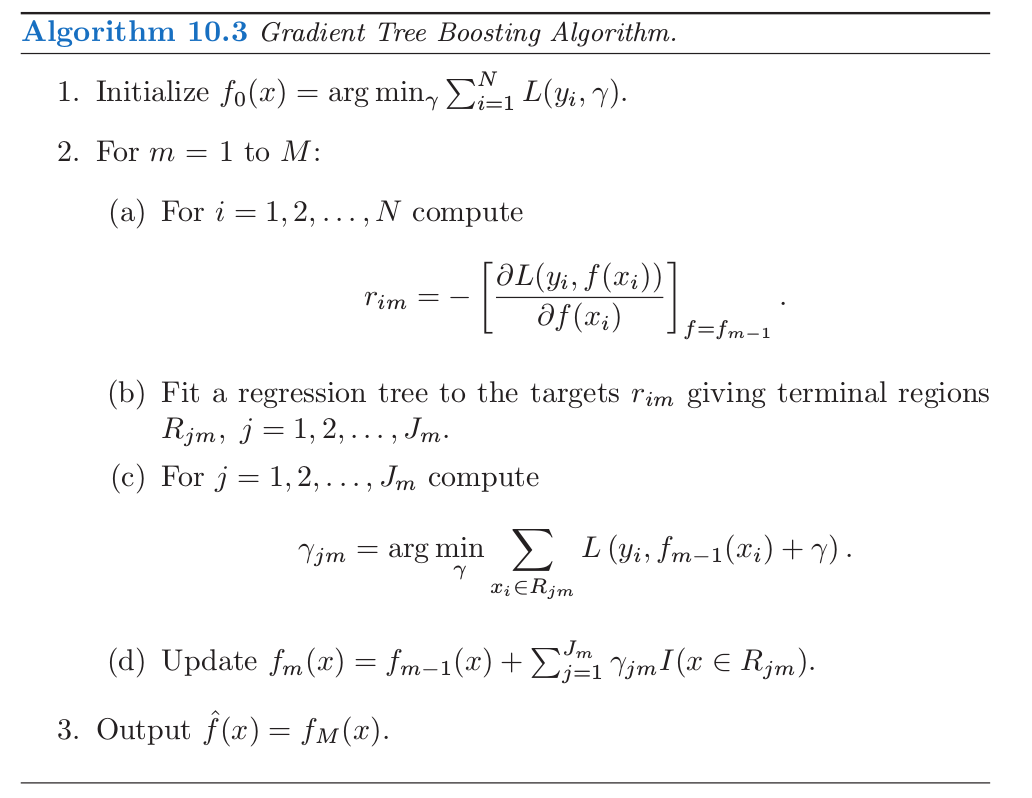“Bet on Sparsity” 原则¶

weiya 注：Ex. 3.6

• 对于给定的应用，稀疏度（稠密度）取决于未知真实函数的个数，以及选定的字典集 $\cal T$．
• 稀疏和稠密的概念相对于训练数据集的大小和（或）噪信比 (NSR)．越大的训练集允许我们估计更小标准差的系数．同样地，在小 NSR 的情形中，当给定样本大小我们能识别出相对于大 NSR 情形下更多的非零系数．
• 字典集的大小也很重要．增大字典集的大小可能导出我们函数的更稀疏的表示，但是寻找问题会变得更困难，导致更高的方差．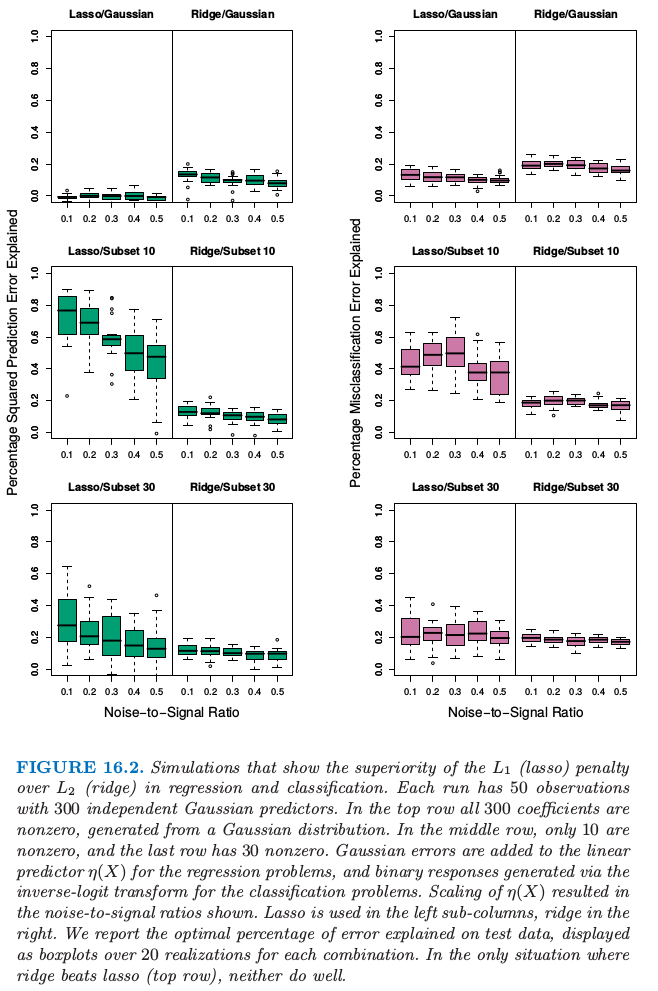weiya 注：

正则化路径，过拟合和 Margin¶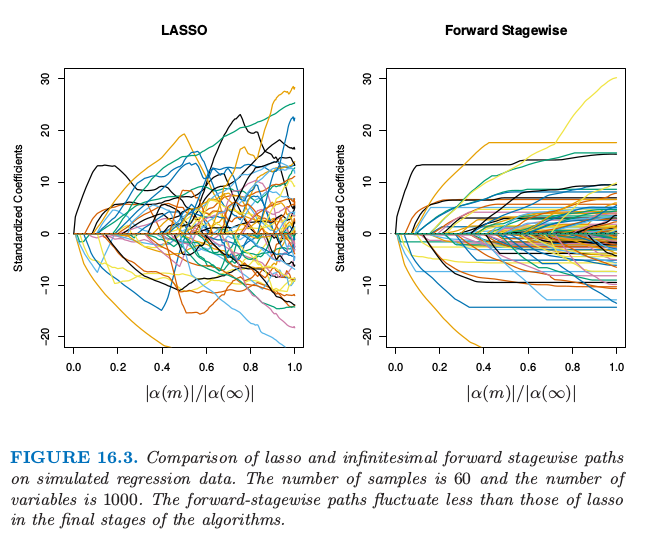weiya 注：Ex. 16.1

$FS_0$ 算法是算法 16.1 的极限形式，其中步长 $\varepsilon$ 收缩到 $0$（3.8.1 节）．变量的分组是想模仿相邻树的相关性，并且向前逐步算法的设定是作为带收缩的梯度加速的理想版本．对于这些算法，系数路径可以精确计算出来，因为他们都是分段线性的（见 3.8.1 节的 LARS 算法）．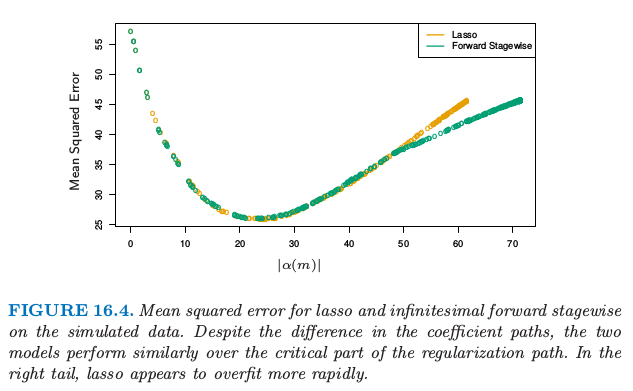Hastie et al. (2007)2 证明了 $FS_0$ 求解了平方误差损失下 lasso 问题的单调版本．令 $\cal T^\alpha=\cal T\cup\{-\cal T\}$ 是通过加入 $\cal T$ 中每个基元素的负值得到的增广字典．我们考虑非负系数 $\alpha_k\ge 0$ 的模型 $f(x)=\sum_{T_k\in {\cal T}^\alpha}\alpha_kT_k(x)$．在增广空间中，lasso 的系数路径是正的，而 $FS_0$ 的则是单调非降的．

weiya 注：Ex. 16.2

Lasso 也可以类似表示成 \eqref{16.6} 式的微分方程的解，不同的是当路径的 $L_1$ 范数增加一个单位是，移动方向会最优地降低损失．结果使得它们不一定是正的，因此 lasso 路径不需要是单调的．

Recall: $L_2$ margin \eqref{4.40}

Schapire et al. (1998)9 证明了对于可分数据，Adaboost 在每步迭代都增加 $m(f)$，收敛到 margin-symmetric 的解．Rätsch and Warmuth (2002)10 证明了带收缩的 Adaboost 渐近收敛到 $L_1$-margin-maximizing 的解．Rosset et al. (2004a)3 考虑对一般损失函数 \eqref{16.2} 形式的正则化模型．他们证明了当 $\lambda\downarrow 0$ 时，对于特定的损失函数，解收敛到 margin-maximizing 的情形．特别地，他们证明 Adaboost 的指数损失属于这种情形，二项偏差也是这种情形．

Boosted 分类器的序列构成 $L_1$ 正则的单调路径，它是某个 margin-maximizing 的解．

1. Efron, B., Hastie, T., Johnstone, I. and Tibshirani, R. (2004). Least angle regression (with discussion), Annals of Statistics 32(2): 407–499.

2. Hastie, T., Taylor, J., Tibshirani, R. and Walther, G. (2007). Forward stagewise regression and the monotone lasso, Electronic Journal of Statistics 1: 1–29.

3. Rosset, S., Zhu, J. and Hastie, T. (2004a). Boosting as a regularized path to a maximum margin classifier, Journal of Machine Learning Research 5: 941–973.

4. Copas, J. B. (1983). Regression, prediction and shrinkage (with discussion), Journal of the Royal Statistical Society, Series B, Methodological 45: 311–354.

5. Donoho, D. and Johnstone, I. (1994). Ideal spatial adaptation by wavelet shrinkage, Biometrika 81: 425–455.

6. Donoho, D. and Elad, M. (2003). Optimally sparse representation from overcomplete dictionaries via l 1 -norm minimization, Proceedings of the National Academy of Sciences 100: 2197–2202.

7. Donoho, D. (2006b). For most large underdetermined systems of equations, the minimal l 1 -norm solution is the sparsest solution, Communications on Pure and Applied Mathematics 59: 797–829.

8. Candes, E. and Tao, T. (2007). The Dantzig selector: Statistical estimation when p is much larger than n, Annals of Statistics 35(6): 2313–2351.

9. Schapire, R., Freund, Y., Bartlett, P. and Lee, W. (1998). Boosting the margin: a new explanation for the effectiveness of voting methods, Annals of Statistics 26(5): 1651–1686.

10. Rätsch, G. and Warmuth, M. (2002). Maximizing the margin with boost- ing, Proceedings of the 15th Annual Conference on Computational Learning Theory, pp. 334–350.

11. Friedman, J., Hastie, T., Rosset, S., Tibshirani, R. and Zhu, J. (2004). Discussion of three boosting papers by Jiang, Lugosi and Vayatis, and Zhang, Annals of Statistics 32: 102–107.